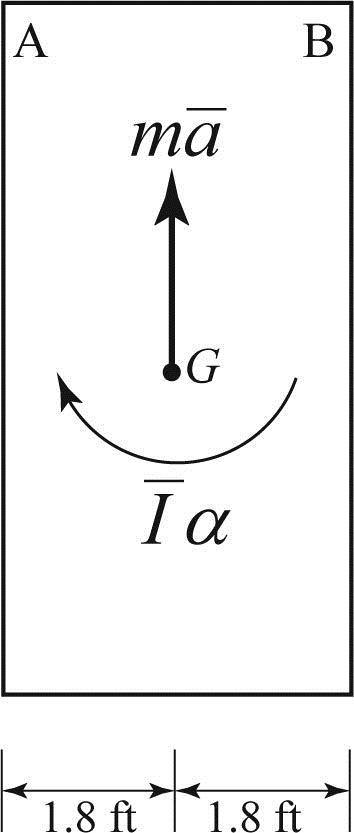# The 400-lb crate shown is lowered by means of two overhead cranes. Knowing the tension in each cable, draw the FBD and KD that can be used to determine the angular acceleration of the crate and the acceleration of the center of gravity. Fig. P16.F4

Question-AnswerCategory: Engineering MechanicsThe 400-lb crate shown is lowered by means of two overhead cranes. Knowing the tension in each cable, draw the FBD and KD that can be used to determine the angular acceleration of the crate and the acceleration of the center of gravity. Fig. P16.F4

The 400-lb crate shown is lowered by means of two overhead cranes. Knowing the tension in each cable, draw the FBD and KD that can be used to determine the angular acceleration of the crate and the acceleration of the center of gravity.
Fig. P16.F4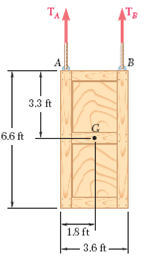Step: 1

The forces acting on the given crate are:
• Tensile force at point A• Tensile force at point B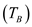• Weight of the crateat center of gravity (G)

Step: 2

Show the free body diagram of the given system as in Figure (1).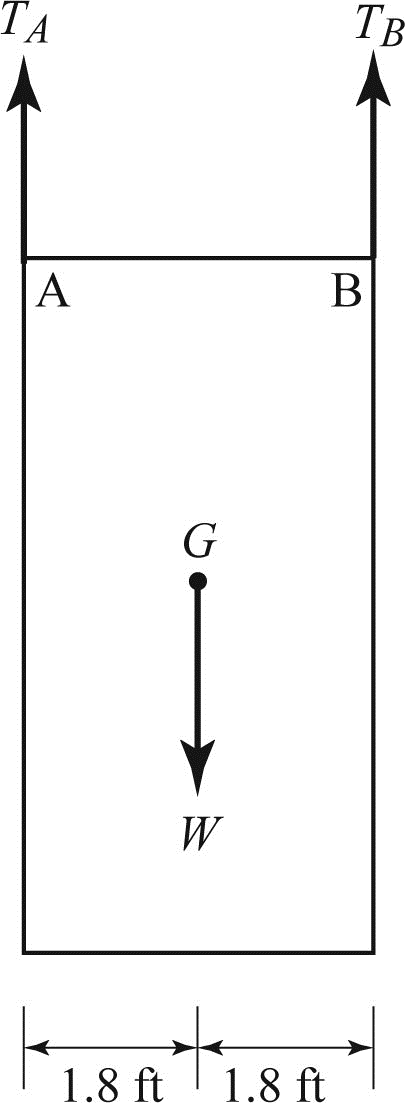• Upward inertial force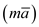at point G